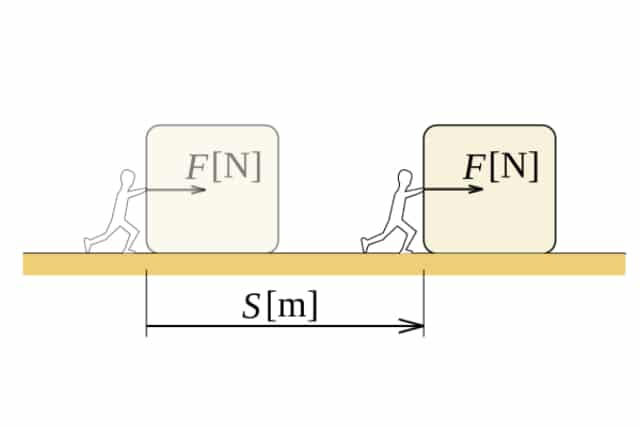In everyday life, the terms “work done” and “power” can mean a lot of things. You may say that you “got a lot of work done today” or “that was a very powerful speech”. However, these words tell a very different story in the field of physics. In this article, we will discuss these two intertwined concepts and present the definitions and formulas that surround them.

Work done

The work done by a force is a measurement of the energy transfer that occurs when a force is applied on an object, causing it to move. In mathematical terms, it is defined as the product of the force being applied on an object, and the distance that it travels in the direction of that force. This distance travelled can also be thought of as a change in position, and hence be referred to as the “displacement”. With this in mind, here is the formula for work done:

• Formula: Work done = Force (N) x Displacement in the direction of the force (m)
• Simplified formula: W = Fs
• SI Unit: Joule (J)

This formula can be applied in a straightforward manner if we know the exact amount of force and resulting displacement. But there are situations where these values must be derived first. One such situation is when we deal with the work done by an expanding gas.

Work done by an expanding gas

We use expanding gases in a bunch of technologies, from pistons in car engines to explosives for demolishing buildings. While you only need to know the final formula for your exams, it is best to understand how it is derived from the base equations.

First, recall the equation for calculating the pressure of gases.

Pressure = Force / Area

Upon rearranging this equation, we get

Force = Pressure x Area

We can then substitute this into our formula for work done, which leaves us with

Work done = Pressure x Area x Displacement

Finally, since Area x Displacement = Change in volume, we get our final formula which describes the work done by an expanding gas against a constant external pressure:

• Formula: Work done by expanding gas = Pressure (Pa) x Change in volume (m3)
• Simplified formula: W = pΔV
• SI Unit: Joule (J)

Power

Power describes the rate of energy conversion. In other words, it is the work done per unit time. Power is an essential measurement because the rate at which you can convert one form of energy to another is just as important as the raw amount of work done.

To visualise this concept, consider car engines. More ‘powerful’ engines would be able to convert the chemical energy in fuel to heat and mechanical energy at a fast rate. This would, in turn, allow the car to travel faster. Power is mathematically defined as the work done divided by the time taken.

• Formula: Power = Work done (J) / Time taken (s)
• Simplified formula: P = W.D. / t
• SI Unit: Watt (W)

Power can also be defined in terms of force and velocity. This equation is derived by simply dividing both sides of the equation for work done by the time taken. After doing so, the equation we are left with is:

Work done / Time taken = Force x (Displacement / Time taken)

Since Work done / Time taken = Power, and Displacement / Time taken = Velocity, we can simplify the formula to:

• Formula: Power = Force (N) x Velocity in the direction of the force (m/s)
• Simplified formula: P = Fv
• SI Unit: Watt (W)

Conclusion

The concepts of work and power are crucial in the field of physics. Knowing how to calculate these measurements in different situations will help immensely in your physics journey, as well as your exams.

If you need any assistance or want to broaden your understanding of the subject, you can engage a reputable physics tuition teacher. Best Physics Tuition holds informative and engaging classes dedicated to helping students excel in O Level and A Level physics.